Blog
Nov 12

# What is a Simulated Treatment Comparison, and can it help my HTA submission?

In this blog we will describe an alternative approach to indirect comparison – Simulated Treatment Comparison (STC) and discuss the key aspects to consider when looking to use the technique. We will discuss the situations when we would recommend using the method instead of a Matching Adjusted Indirect Comparison (MAIC).

HTA submissions often involve demonstrating the relative efficacy or safety of one treatment compared to one or more competitor treatments. Direct comparisons between trial outcomes when using standard network meta-analyses (NMA) rely on the assumption that there is no difference between trials in the distribution of trial-level (marginal) effect modifiers. Put simply, we assume that the characteristics of any two trial populations are the same, in all the ways that would affect the relative benefits of the treatments for the outcome of interest.

In practice, this is unlikely to be the case. In earlier blogs we have introduced the concept of population adjustment to account for differences between trials that may have an impact on the absolute outcome (prognostic variables) or relative treatment effect (effect modifying variables). We have also outlined one approach to population adjustment – Matching Adjusted Indirect Comparison (MAIC).

STC involves estimating a linear regression model for the relationship between population characteristics and outcome in a trial where individual patient data is available, and then using the model to estimate that outcome for the other trial population.

### Setting

We will be considering an unanchored direct comparison between two treatments. ‘Unanchored’ in this sense means that there is no third treatment (e.g. placebo) that both treatments of interest have been compared to.

Imagine a drug company has recently developed and trialled a new treatment A, where the trial demonstrating efficacy was single-armed (e.g.  for ethical reasons). This company wishes to make a (hopefully favourable!) comparison to a competitor’s treatment, B, for which the published data of a single-armed trial is available. In each trial, a different treatment has been evaluated with the purpose of keeping the value of a biomarker as low as possible. Whilst the company has the individual patient data (IPD) for the A trial, only the summary statistics  of the population characteristics in the B trial are available.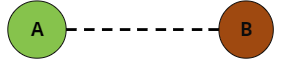Our aim is to make a comparison between A and B. Ideally, we want to estimate the difference between treatment outcomes of A and B in some target population P. In practice, we will estimate the difference between outcomes for A and B on the population of the B trial; this makes for easier maths, and fewer assumptions.

### Analyse the Data

First, let’s analyse how the data for the trial where we have IPD (trial A) compares to the summary statistics for the trial B where we do not have IPD. The figure below shows the distributions of four covariates from trial A (in green) compared with the summary statistics of each for trial B (brown dotted lines).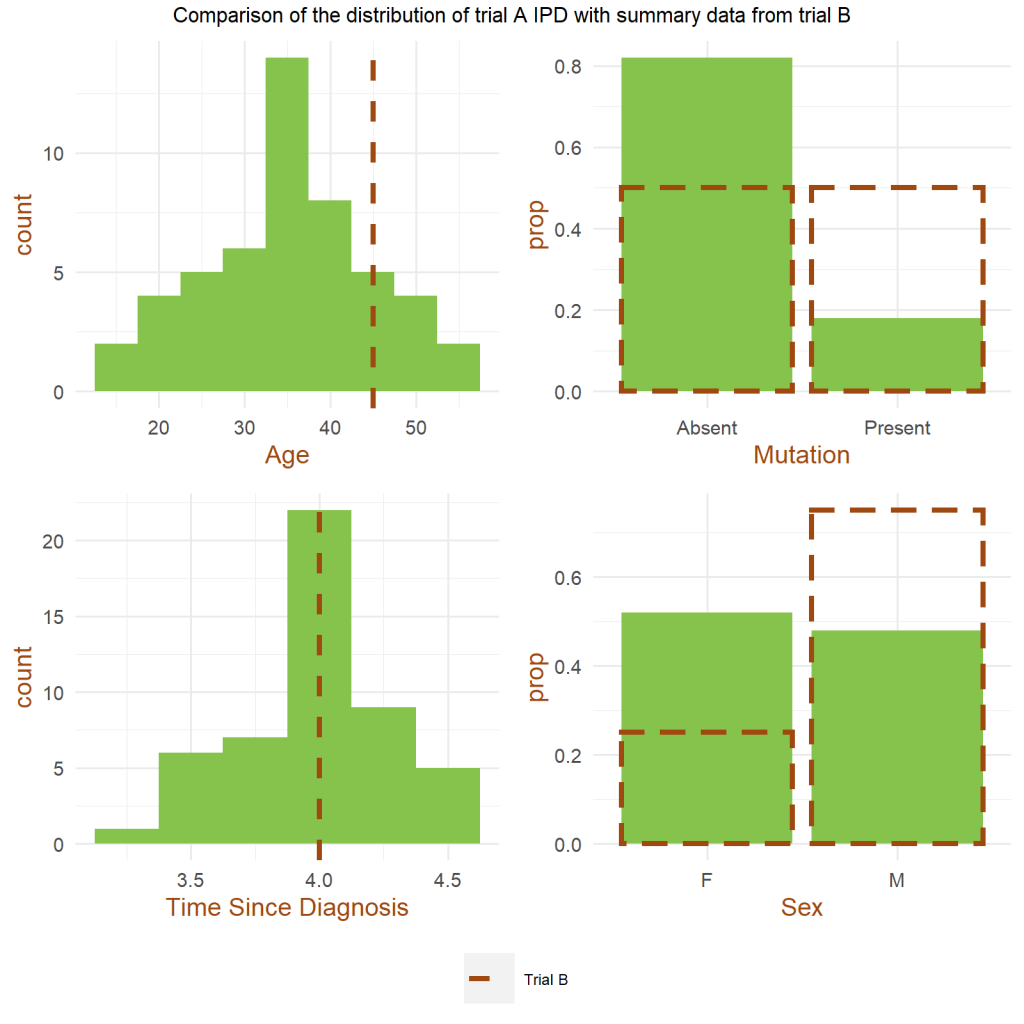It’s clear that trial B was performed on a population quite dissimilar from that in trial A. Other than Time Since Diagnosis, where the reported mean for trial B is close to the mean for trial A, the covariate distributions  are clearly different. In addition, we should exercise caution when stating that the populations were similar with respect to Time Since Diagnosis; the mean being in the same place tells us nothing about the shape of the distribution of participants in trial B. For example, if the true distribution on Time Since Diagnosis for trial B were bimodal, the populations would be substantially different, despite having similar means.

However, we do have sufficient evidence to suggest that directly comparing the effects of the treatments from each trial is inappropriate. The trial populations are too dissimilar for us to be confident that the raw relative treatment effect is due only to difference in administered treatment. Any of the above covariates might be an effect modifier or a prognostic variable, and as such would need to be accounted for before we can make a comparison of treatment effect.

For clarity in this blog, we’re only going to focus on the relationship between one covariate and the biomarker we are using to measure effect. Let’s plot the correlations between all the covariates and the biomarker to see which covariate is most likely to modify the effect.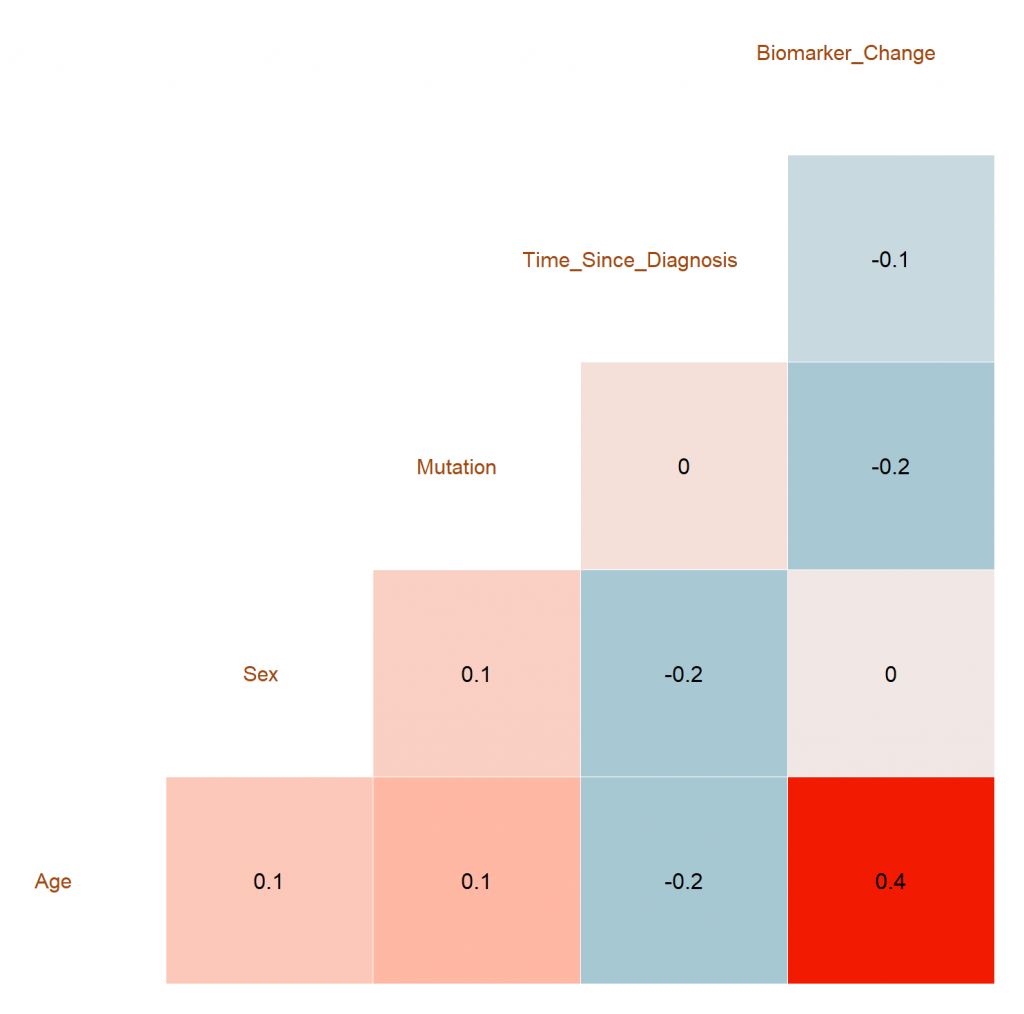Age has the strongest correlation with the change recorded in the effect biomarker, so to illustrate STC, we will concentrate on the relationship between age and the trial outcome (change in the relevant biomarker).

### Simulated Treatment Comparison (STC)

The outcome of any trial is dependent on the treatment provided and the population the treatment is provided to. Trials A and B have provided us with an estimate of the expected outcome of treatment A on the population of trial A, and treatment B on the population of trial B. However, to perform a comparison, we either need an estimate of the expected outcome of treatment A on the population trial B, or that of treatment B on the population of trial A. STC achieves this estimate by learning a linear model relating covariates to outcomes.

To do this we fit an outcome model for treatment A using the trial for which we have IPD (trial A). Once the model of outcomes for treatment A has been fitted to our satisfaction, we can use it to estimate the outcome of treatment A on the population of trial B.

{Mathematical detail:

This model  can be written using the following mathematical notation:

$$g(\mu($$ $$X_A$$$$)) = \beta_0 + \beta_1 *$$ $$X_A$$

Here $$X_A$$ is the vector of covariate values corresponding to a particular individual in trial A. $$\mu($$$$X_A$$$$)$$ is the outcome of the trial for that individual, and $$\beta_0$$ and $$\beta_1$$ are the parameters of the model, which we estimate using the data for all the individuals in trial A.  Here, because we’re only considering age, $$X_A$$ will just be the age of each participant.

$$g$$ is a  “link function” which converts between the scale we use when fitting the regression model (e.g. log hazards ratio) and the outcome scale (e.g. hazard ratio). We do this because it is often the case that a linear model, like the one above, won’t easily fit the outcomes directly. The link function lets us transform the outcomes to a scale that the linear model works well in. Here, we’re going to assume $$g$$ is the identity function, so it doesn’t change anything.

The model of outcomes for treatment A can now be used  to estimate the outcome of treatment A on the population of trial B  by substituting in the mean covariate values of trial B, which in this case is the mean age of participants in population B, $$\bar{X}_B$$:

$$g(\mu($$ $$\bar{X}_B$$$$)) = \beta_0 + \beta_1 *$$ $$\bar{X}_B$$

}

The plot below shows the results of fitting the regression model between age and biomarker change graphically.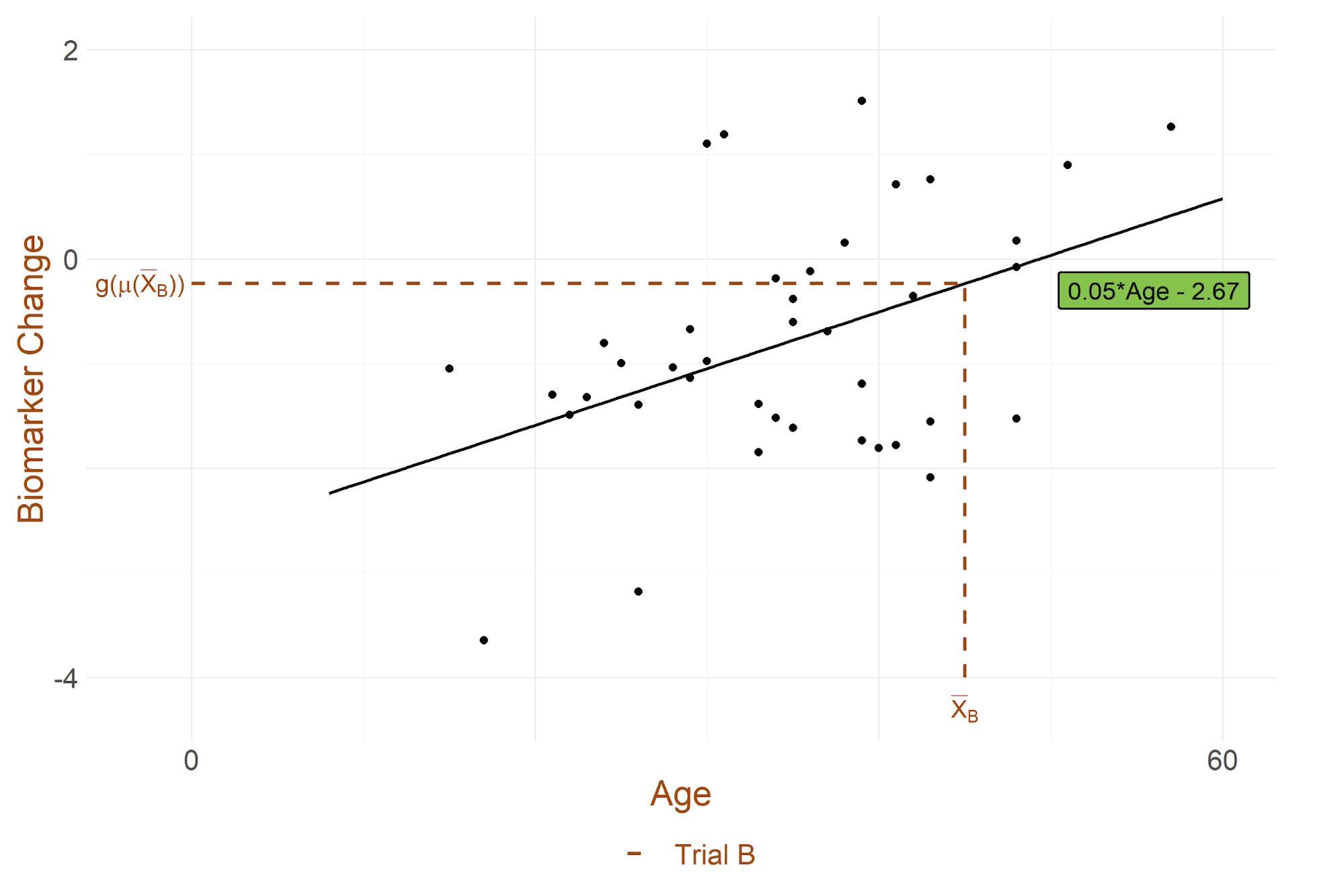The black line is the estimated linear relationship for treatment A between age and outcome (the change in the biomarker), {with $$\beta_0 = -2.67$$ and $$\beta_1 = 0.05$$}.  The  mean age of participants in B (45 years) directly predicts a mean biomarker change of -0.23 for treatment A when applied to the B population.

As the mean biomarker change for treatment B on the B population was -0.45, we can now say that the difference between treatment A and treatment B is a mean biomarker change of -0.22. In other words, if negative biomarker change is a favourable outcome, treatment B is an improvement on treatment A by a relative change of -0.22,  if both treatments are given to  the B population.

### Assumptions and Caveats

Firstly, we should note that a one-dimensional model is not particularly powerful; ideally we would fit a multivariate linear model to as many covariates as we have available (and this is also what NICE and other regulatory agencies would require). In general, STC also estimates different sets of parameters for those covariates which are solely prognostic compared to those which are also effect-modifying.

We have already noted that by fitting a linear model, we are making strong assumptions about the relationship between covariates and outcome. The link function attempts to mitigate this by allowing us to transform to a scale where we can assume effects are additive and linear, however because our estimates are made in the transformed scale, we should also perform our comparison of treatments on this scale, before transforming back to the real outcome scale.

Fitting a linear model also comes with its own collection of assumptions; normally it is sensible to hold out some data to get an estimate of how well the model will generalise to unseen data (the model is an estimate of behaviour on the full population of interest, so caution should be taken not to ‘overfit’ to the sample of participants we actually have).

An estimate of how well the model is performing on the data it was trained on is also a useful thing to check; if the treatment A model has a large error on the population of trial A, this variance will carry over and further limit the conclusions that can be drawn about the efficacy of treatment A on the population of trial B. Below we plot the residuals (errors) of our model on the held-out set of individuals from population A. An average of these errors is a good first hint of whether the model is good enough for the indirect comparison to continue. In this case, the root mean squared error of prediction is 1.07, which is comparable to the estimated benefit of B vs A. This means that the estimated benefit could equally well reflect a prediction error as reflect a “true” benefit.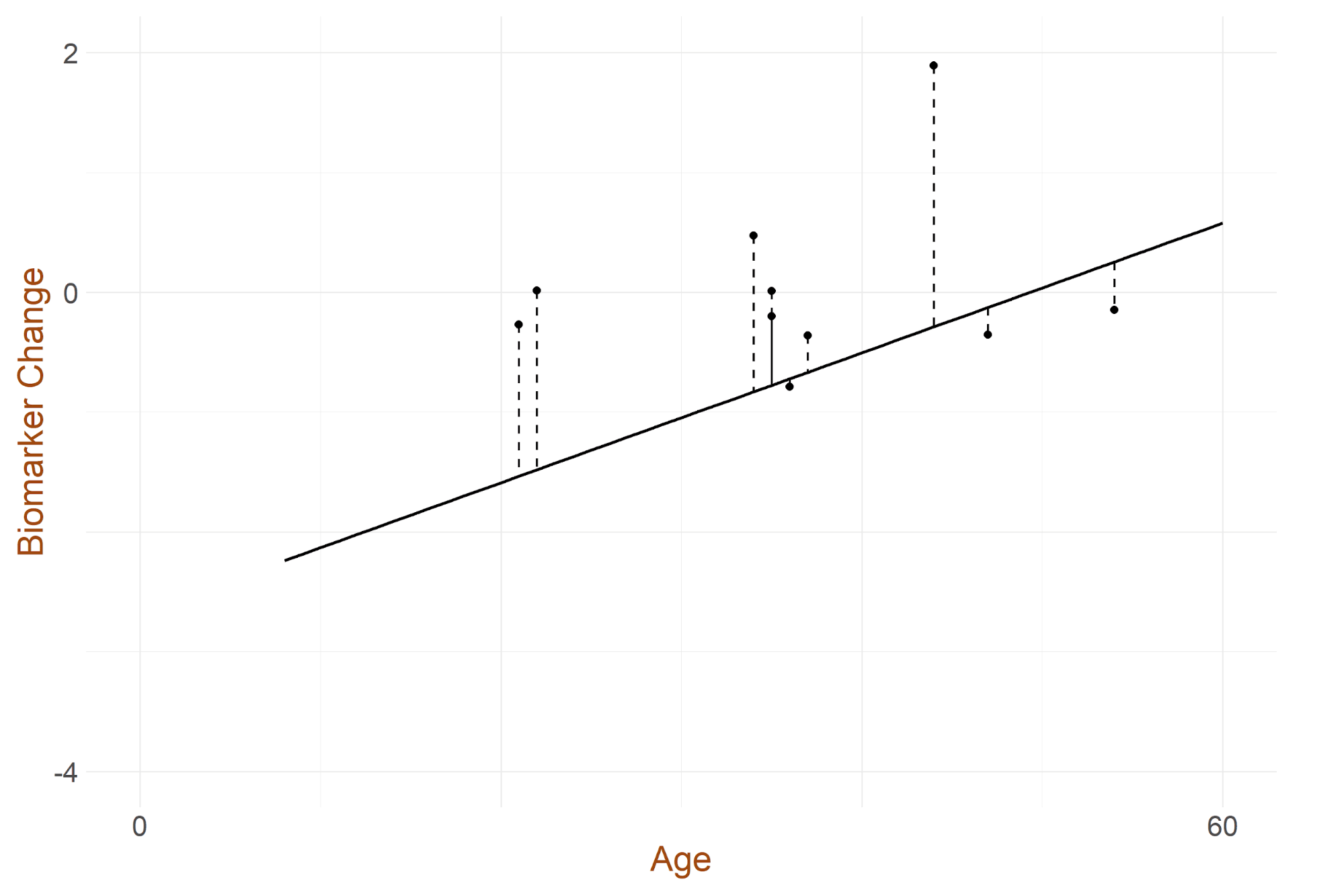Along similar lines, the trial we have the IPD for should have been correctly randomised; we need the sample to be random enough for us to treat it as an unbiased draw from the underlying true covariate distribution. This allows us to assume within-study covariate balance.

In addition, we should be cautious about predictions our model makes in areas where we have seen no data, or very little data. If the mean age for a participant in B was 75, for example, when every participant we’re using to fit our model is below 55, we should have to be very cautious about making claims based on our linear model.

The NICE documentation on MAIC/STC provides 5 recommendations when considering using STC or similar:

1. Anchored comparison may be considered when there is connected evidence with a common comparator. Unanchored comparisons may only be considered where single-arm studies are involved, or in the absence of a connected network of randomised evidence.
2. Submissions using anchored population adjusted analyses need to provide evidence that they are less likely to produce biased estimates of treatment difference than could be achieved through standard methods, and that population adjustment would have a material impact on relative effect estimates due to the removal of substantial bias.
3. Submissions using unanchored population adjusted analyses need to provide evidence that absolute outcomes can be predicted with sufficient accuracy in relation to the relative treatment effects. Our plotting of the residual errors, above, is an example of this.
4. Outcome regression methods should adjust for all effect modifiers and any prognostic variables that improve model fit in anchored comparisons. In unanchored comparisons, all effect modifiers and prognostic factors should be adjusted for, in order to reliably predict absolute outcomes.
5. Indirect comparisons should be carried out on the linear predictor scale, as discussed above.

### Conclusion

We have given an overview of a second way to approach Indirect Model Comparison in NMA – Simulated Treatment Comparison or STC. STC is one way to compare treatments when attempting to claim relative efficacy or safety in a HTA submission.

Here, we have presented an unanchored comparison but STC can also be used to make anchored comparisons, for example when both treatments A and B are compared to a further treatment C (such as placebo). The maths in this case is a little more convoluted, but the underlying approach is the same.

One main difference between MAIC and STC is that the MAIC algorithm can fail to evenly distribute weights across the participants of the trial (see the MAIC blog for details). On the other hand, STC will always provide an estimate of relative efficacy or safety. However, as the reason MAIC fails is generally due to substantial dis-similarity between the two trials of interest, the STC estimate should be examined to check it is reasonable.

By virtue of fitting a model to the relationship between covariates and outcomes, STC has the potential to generalise beyond the range of the IPD seen in the IPD trial. It also provides an insight into how covariates relate to outcomes, which can be useful if it is necessary to justify the relative treatment comparison. As with any indirect comparison, caution should be exercised.  Results should be presented with robust caveats as to their likely scope, and consequently weighed alongside expert clinical opinion and additional evidence before decisions are made.

Recent work has suggested a more general approach to indirect treatment comparison, which can be used to compensate for the weaknesses of both MAIC and STC. This is Multilevel Network Meta-Regression (ML NMR), and will be the topic of a future blog.

### Be First In Line For New Content

By Checking the above you accept we may send you relevant information by email.

The NICE view of MAIC/STC: http://nicedsu.org.uk/wp-content/uploads/2018/08/Population-adjustment-TSD-FINAL-ref-rerun.pdf, contains a good number of cautions.

A paper by one of the inventors of STC on both STC and MAIC: https://pubmed.ncbi.nlm.nih.gov/25795232/

A paper which uses STC to perform an indirect treatment comparison:

https://www.ncbi.nlm.nih.gov/pmc/articles/PMC4245493/pdf/12325_2014_Article_167.pdf

A paper which uses STC to perform an indirect treatment comparison: